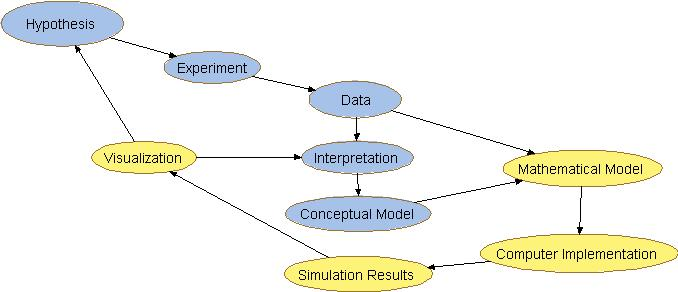## The Process of Modeling

The process of developing the mathematical models can be described by the steps listed below.  These are the steps that occur after experimentation and a base level of understanding has been obtained. A concept map of the biological research process that includes mathematical modeling (yellow) is also shown. The "traditional" biological research process is shown in blue.

 1. List components of the biological system 2. List interactions between components 3. Create concept map 4. Transcribe map into mathematical equations 5. Implement model in computer code/ software 6. Simulate 7. Visualize and InterpretAdditional Introductory Materials for Modeling

Wofford College: Computational Science Emphasis for Undergraduates
Scinetific Computing, Modeling

Robert Phair's Integrative Bioinformatics:
Practical Kinetic Modeling of Large Scale Biological Systems

Herbert Sauro's Intro to Modeling using JDesigner.